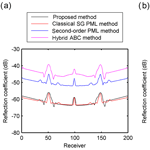Solid Earth, 9, 1277–1298, 2018
https://doi.org/10.5194/se-9-1277-2018

Special issue: Advances in seismic imaging across the scales

Solid Earth, 9, 1277–1298, 2018
https://doi.org/10.5194/se-9-1277-2018

Research article 07 Nov 2018

Research article | 07 Nov 2018# Second-order scalar wave field modeling with a first-order perfectly matched layer

Xiaoyu Zhang, Dong Zhang, Qiong Chen, and Yan Yang Xiaoyu Zhang et al.
• School of Physics and Technology, Wuhan University, Wuhan, Hubei, China

Abstract. The forward modeling of a scalar wave equation plays an important role in the numerical geophysical computations. The finite-difference algorithm in the form of a second-order wave equation is one of the commonly used forward numerical algorithms. This algorithm is simple and is easy to implement based on the conventional grid. In order to ensure the accuracy of the calculation, absorption layers should be introduced around the computational area to suppress the wave reflection caused by the artificial boundary. For boundary absorption conditions, a perfectly matched layer is one of the most effective algorithms. However, the traditional perfectly matched layer algorithm is calculated using a staggered grid based on the first-order wave equation, which is difficult to directly integrate into a conventional-grid finite-difference algorithm based on the second-order wave equation. Although a perfectly matched layer algorithm based on the second-order equation can be derived, the formula is rather complex and intermediate variables need to be introduced, which makes it hard to implement. In this paper, we present a simple and efficient algorithm to match the variables at the boundaries between the computational area and the absorbing boundary area. This new boundary-matched method can integrate the traditional staggered-grid perfectly matched layer algorithm and the conventional-grid finite-difference algorithm without formula transformations, and it can ensure the accuracy of finite-difference forward modeling in the computational area. In order to verify the validity of our method, we used several models to carry out numerical simulation experiments. The comparison between the simulation results of our new boundary-matched algorithm and other boundary absorption algorithms shows that our proposed method suppresses the reflection of the artificial boundaries better and has a higher computational efficiency.

Share